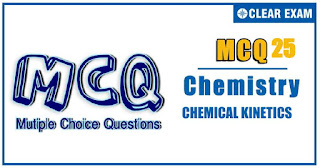## [LATEST]\$type=sticky\$show=home\$rm=0\$va=0\$count=4\$va=0

IIT JEE exam which consists of JEE Main and JEE Advanced is one of the most important entrance exams for engineering aspirants. The exam is held for candidates who are aspiring to pursue a career in the field of engineering and technical studies. Chemistry is important because everything you do is chemistry! Even your body is made of chemicals. Chemical reactions occur when you breathe, eat, or just sit there reading. All matter is made of chemicals, so the importance of chemistry is that it's the study of everything..

Q1.Statement 1: Temperature coefficient is the ratio of two rate constants preferably at 35℃ and 25℃. Statement 2: It can also be given as e^(-E_a/R [(T_2-T_1)/(T_1 T_2 )] )
•  Statement 1 is True, Statement 2 is True; Statement 2 is correct explanation for Statement 1
•  Statement 1 is True, Statement 2 is True; Statement 2 is not correct explanation for Statement 1
•  Statement 1 is True, Statement 2 is False
•  Statement 1 is False, Statement 2 is True
Solution
(d) Both statement and explanation are correct but the reason is different

Q2.Statement 1: Photosynthesis in plants involves reaction of CO_2 and H_2 O in presence of light and chlorophyll. Statement 2: It is chlorophyll which absorbs light and passes this energy to reactant molecules. :
•  Statement 1 is True, Statement 2 is True; Statement 2 is correct explanation for Statement 1
•  Statement 1 is True, Statement 2 is True; Statement 2 is not correct explanation for Statement 1
•  Statement 1 is True, Statement 2 is False
•  Statement 1 is False, Statement 2 is True
Solution
(a) Nacl has fcc structure in which each Na^+ is surrounded by six ions and vic versa.In this octahedral arrangement, coordination, number of bothNa^+ and Cl^- is six for which radius ratio lies between 0.414 and 0.732. The radius ratio does not allow Cl^- ions to touch each other.

Q3.  Statement 1: Rate constant K is equal to Arrhenius parameter if it involves free radical combination. Statement 2: E_a=0 for free radical combination.
•  Statement 1 is True, Statement 2 is True; Statement 2 is correct explanation for Statement 1
•  Statement 1 is True, Statement 2 is True; Statement 2 is not correct explanation for Statement 1
•  Statement 1 is True, Statement 2 is False
•  Statement 1 is False, Statement 2 is True
Solution
(c) Explanation is correct reason for statement.

Q4. Statement 1: For the reaction RCl+NaOH(aq)→ROH+NaCl, the rate of reaction is reduced to half on reducing the concentration of RCl to half Statement 2: The rate of the reaction is represented by k[RC], i.e., it is a first order reaction
•  Statement 1 is True, Statement 2 is True; Statement 2 is correct explanation for Statement 1
•  Statement 1 is True, Statement 2 is True; Statement 2 is not correct explanation for Statement 1
•  Statement 1 is True, Statement 2 is False
•  Statement 1 is False, Statement 2 is True
Solution
(a) Packing fraction in fcc = 74% Packing fraction in bcc = 67.9%

Q5.Statement 1: As the temperature increases, rate of reaction increase Statement 2: The rate of reaction increase due to, increase in collision frequency and shortening of mean free path
•  Statement 1 is True, Statement 2 is True; Statement 2 is correct explanation for Statement 1
•  Statement 1 is True, Statement 2 is True; Statement 2 is not correct explanation for Statement 1
•  Statement 1 is True, Statement 2 is False
•  Statement 1 is False, Statement 2 is True
Solution
(d) Both statement and explanation are correct but reason for the statement is different

Q6Statement 1: The rate of reaction sometimes does not depend on concentration Statement 2: The order of reaction can be negative
•  Statement 1 is True, Statement 2 is True; Statement 2 is correct explanation for Statement 1
•  Statement 1 is True, Statement 2 is True; Statement 2 is not correct explanation for Statement 1
•  Statement 1 is True, Statement 2 is False
•  Statement 1 is False, Statement 2 is True
Solution
(a) For fcc, Number of O^(2-) ions =8(corners)×1/8per corner share +6 (faces)×1/2per face share=4 Number of Si^(4+) ions =1/4×OV=1/4×4=1/unit cell Number Fe^(2+) ions =1/4×TV=1/4×8=2/unit cell ⇒Fe_2 SiO_4

Q7. Statement 1: The rate of reaction whether exothermic or endothermic, increases with temperature. Statement 2: The rate of reaction =K[reactant]^n and K increases with temperature.
•  Statement 1 is True, Statement 2 is True; Statement 2 is correct explanation for Statement 1
•  Statement 1 is True, Statement 2 is True; Statement 2 is not correct explanation for Statement 1
•  Statement 1 is True, Statement 2 is False
•  Statement 1 is False, Statement 2 is True
Solution

Q8.Statement 1: k∝e^(-E¬_a/RT) the Arrhenius equation represents the dependence of rate constant with temperature Statement 2: Plot of log⁡k against 1/T is linear and the activation energy can be calculated with this plot
•  Statement 1 is True, Statement 2 is True; Statement 2 is correct explanation for Statement 1
•  Statement 1 is True, Statement 2 is True; Statement 2 is not correct explanation for Statement 1
•  Statement 1 is True, Statement 2 is False
•  Statement 1 is False, Statement 2 is True
Solution
(c) The distance 0.204 nm comes out to be " a⁄√3 " which corresponds to the perpendicular distance between a corner of unit cell and the plane of the three adjacent corners (Fig. III)#### Written by: AUTHORNAME

AUTHORDESCRIPTION## Want to know more

Please fill in the details below:

Name